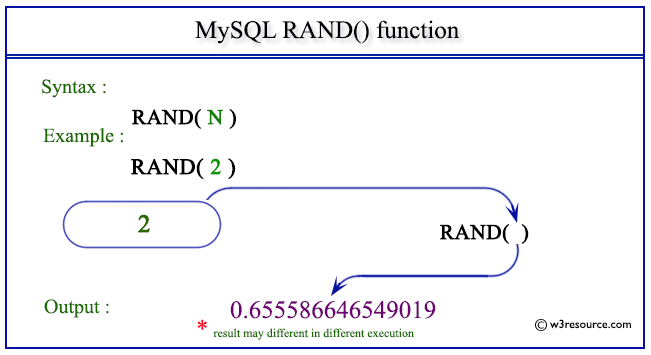# MySQL RAND() function

## RAND() function

MySQL RAND() returns a random floating-point value between the range 0 to 1. When a fixed integer value is passed as an argument, the value is treated as a seed value and as a result, a repeatable sequence of column values will be returned.

This function is useful in -

• RAND() is crucial for tasks that require random sampling from a dataset.
• In software development, RAND() is used to perform randomized testing.
• RAND() is used to create unpredictable behavior, making games more dynamic and realistic.
• RAND() can be used to generate random keys, salts, and initialization vectors.
• In web applications, RAND() can be used to personalize content. For instance, a website might display different featured articles to different users on each visit.
• In scientific research, RAND() is used to simulate scenarios for statistical analysis.
• In scenarios where a system needs to be stress-tested, random inputs can be generated using RAND().

Syntax:

```RAND(), RAND(M);
```

Argument:

Name Description
M A number between 0 to 1.

MySQL Version: 8.0

Pictorial presentation of MySQL RAND() functionExample of MySQL RAND() function

Code:

``````SELECT RAND();
```
```

Explanation:

The above MySQL statement will return a random value between 0 and 1.

Output:

```mysql> SELECT RAND();
+-------------------+
| RAND()            |
+-------------------+
| 0.369500624360052 |
+-------------------+
1 row in set (0.00 sec)
```

Example: RAND() function with seed value

Code:

``````SELECT RAND(),RAND(2),RAND(2);
```
```

Explanation:

The above MySQL statement will return a random value (between 0 and 1) and the repeatable value using seed in the argument.

Output:

```mysql> SELECT RAND(),RAND(2),RAND(2);
+-------------------+-------------------+-------------------+
| RAND()            | RAND(2)           | RAND(2)           |
+-------------------+-------------------+-------------------+
| 0.964232316207357 | 0.655586646549019 | 0.655586646549019 |
+-------------------+-------------------+-------------------+
1 row in set (0.00 sec)
```

Example: RAND() function using table

Code:

``````SELECT FLOOR(RAND()*10)
FROM category;
```
```

Explanation:

The above MySQL statement will return the largest integer value after multiply the randomly generated number by 10 but not greater than the generated number.

Output:

```mysql> SELECT FLOOR(RAND()*10)
->   FROM category;
+------------------+
| FLOOR(RAND()*10) |
+------------------+
|                7 |
|                6 |
|                2 |
|                0 |
|                6 |
+------------------+
5 rows in set (0.29 sec)
```

Example: RAND() function with where clause

Code:

``````SELECT pub_name,country,no_of_branch,
FLOOR(RAND(2)*20)
FROM publisher
WHERE no_of_branch>FLOOR(RAND(2)*20);
```
```

Explanation:

The above MySQL statement will return those rows from publisher table which have no_of_branchs are more than the greatest number after generating the random number with FLOOR function.

Output:

```mysql> SELECT pub_name,country,no_of_branch,
->    FLOOR(RAND(2)*20)
->       FROM publisher
->     WHERE no_of_branch>FLOOR(RAND(2)*20);
+--------------------------+---------+--------------+-------------------+
| pub_name                 | country | no_of_branch | FLOOR(RAND(2)*20) |
+--------------------------+---------+--------------+-------------------+
| Jex Max Publication      | USA     |           15 |                13 |
| BPP Publication          | India   |           10 |                 2 |
| Mountain Publication     | USA     |           25 |                12 |
| Summer Night Publication | USA     |           10 |                17 |
| Novel Publisher Ltd.     | India   |           10 |                 7 |
+--------------------------+---------+--------------+-------------------+
5 rows in set (0.13 sec)
```

All Mathematical Functions